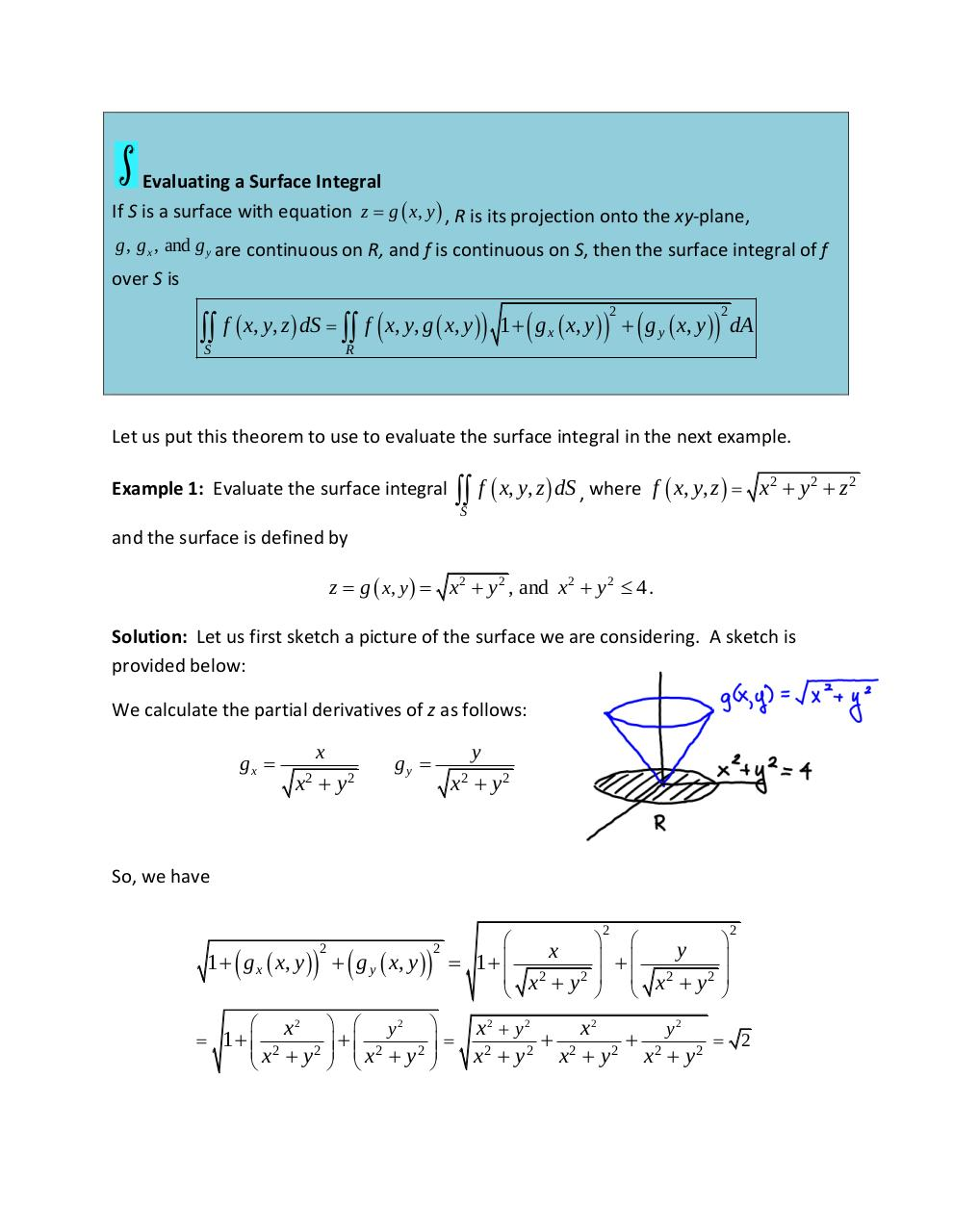# Lesson 10 6 Surface Integrals.pdfPage 1 2 3 4 5 6 7

#### Text preview

Evaluating a Surface Integral
If S is a surface with equation z  g  x, y  , R is its projection onto the xy-plane,
g , g x , and g y are continuous on R, and f is continuous on S, then the surface integral of f

over S is

 f  x, y, z  dS   f  x, y, g  x, y  1   g x  x, y    g y  x, y  dA
2

S

2

R

Let us put this theorem to use to evaluate the surface integral in the next example.

S f  x, y, z  dS , where f  x, y, z  

Example 1: Evaluate the surface integral

x2  y 2  z 2

and the surface is defined by

z  g  x, y   x2  y 2 , and x2  y 2  4 .
Solution: Let us first sketch a picture of the surface we are considering. A sketch is
provided below:
We calculate the partial derivatives of z as follows:

gx 

x

x2  y 2

y

gy 

x2  y 2

So, we have
2

1   g x  x, y     g y  x, y  
2

 x2
 1  2
 x  y2

  y2
 2
  x  y2
 

2

y
x
 

 1 
 x2  y 2 
 x2  y 2 



2

x2  y 2
x2
y2

 2
x2  y 2 x2  y 2 x2  y 2